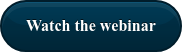# Pt.1 - How to assess the insulation of a transformer with tan delta testing

## Why is Tan Delta testing so important to assess the insulation of a Transformer? Let's find out how to perform Tan Delta test and how to read results with ISA test equipment### Why do we perform tan delta test?

We perform tan delta test to define the state of insulation of the asset or to find out how far we are from the insulation faults. Since insulation has a very non-linear characteristic, a very small increase in resistive current can suddenly make the insulation break. It is important to say that high value (if not too high) is not necessarily a sign that we need to change the asset. Often, we just need to follow the trend and operate if the change is too big.

IEEE defines electrical insulation as “material or a combination of suitable non-conducting materials that provide electrical isolation of two parts at different voltages”.

The most common technique used to assess the insulation of primary equipment like a transformer, rotating machine, cables, circuit breaker, is an AC test.

AC test is used to check the aging of the insulation and possible mechanical deformation.

What kind of electrical insulation is used today?

• Solid: Cellulose (Paper or Pressboard), cast resin, porcelain
• Liquid: mineral oils, silicone oils, synthetic compounds
• Gaseous: Sulphur Hexafluoride (SF6), air and vacuum, nitrogen
A pure insulator acts like a capacitor, when it is connected across line and earth. In an ideal insulation system, as the insulating material which acts as dielectric too, is 100 % pure, the electric current passing through the insulator, only have capacitive component. There is no resistive component of the current, flowing from line to earth through insulator as in ideal insulating material. In a pure capacitor, the capacitive electric current leads the applied voltage by 90o. Practically, the insulator cannot be manufactured 100% pure and also the dirt and moisture provide the conductive path to the current. Hence, an electric leakage current flowing from line to earth through the insulator has a resistive component.

For good insulator, this resistive component of electric leakage current must be low. So, the health condition of an electrical insulator can be determined by the ratio of the resistive component to the capacitive component. This ratio is known as “tan delta”, sometimes also defined “dissipation factor”.In the vector scheme: x-axis represents the voltage system and the resistive component of leakage current, IR.

As the capacitive component of leakage electric current IC leads system voltage by 90o, it will be drawn along the y-axis.

Total leakage electric current IL(Ic + IR) makes an angle δ with y-axis.

The ratio, IR to IC is nothing but tanδ or tan delta.### Method of Tan Delta Testing

First, the normal voltage is applied. If the value of tan delta looks good enough, the voltage is raised to 1.5 to 2 times of normal voltage, of the asset. A that point, the testing unit takes measurement of tan delta values and compares the tan delta values at normal voltage and higher voltages and analyze the results.

During the test, it is essential to apply test voltage at a very low frequency, in order to keep the power requirement for this dissipation factor test. The frequency range for tan delta test is generally from 0.1 to 0.01 Hz depending upon size and nature of insulation.### How to read the result of tan delta testing

There are two ways of reading the condition of an insulation system during a tan delta test.

The first one, is to compare the results of previous tests, to determine the deterioration of the insulation due to aging effects. While, the second one, is to determine the condition of insulation from the value of tan delta, directly. If the insulation is perfect, the loss factor will be approximately the same for all range of test voltages. But if the insulation is not enough, the value of tan delta increases in the higher range of test voltage.

### How do we perform tan delta test?

ISA - ALTANOVA Group offers 2 different solutions for testing the insulation of a transformer.

•         STS 5000 + TD 5000: for a complete substation commissioning and maintenance
•         TDX 5000: a compact solution for high voltage Capacitance and Tan Delta testing